Puzzle | Weight of Heavy Ball

• Difficulty Level : Easy
• Last Updated : 17 Feb, 2020

Puzzle: There are 2187 balls, out of which 1 is heavy. Find the minimum number of attempts the balls have to be weighed for finding out the heavy ball.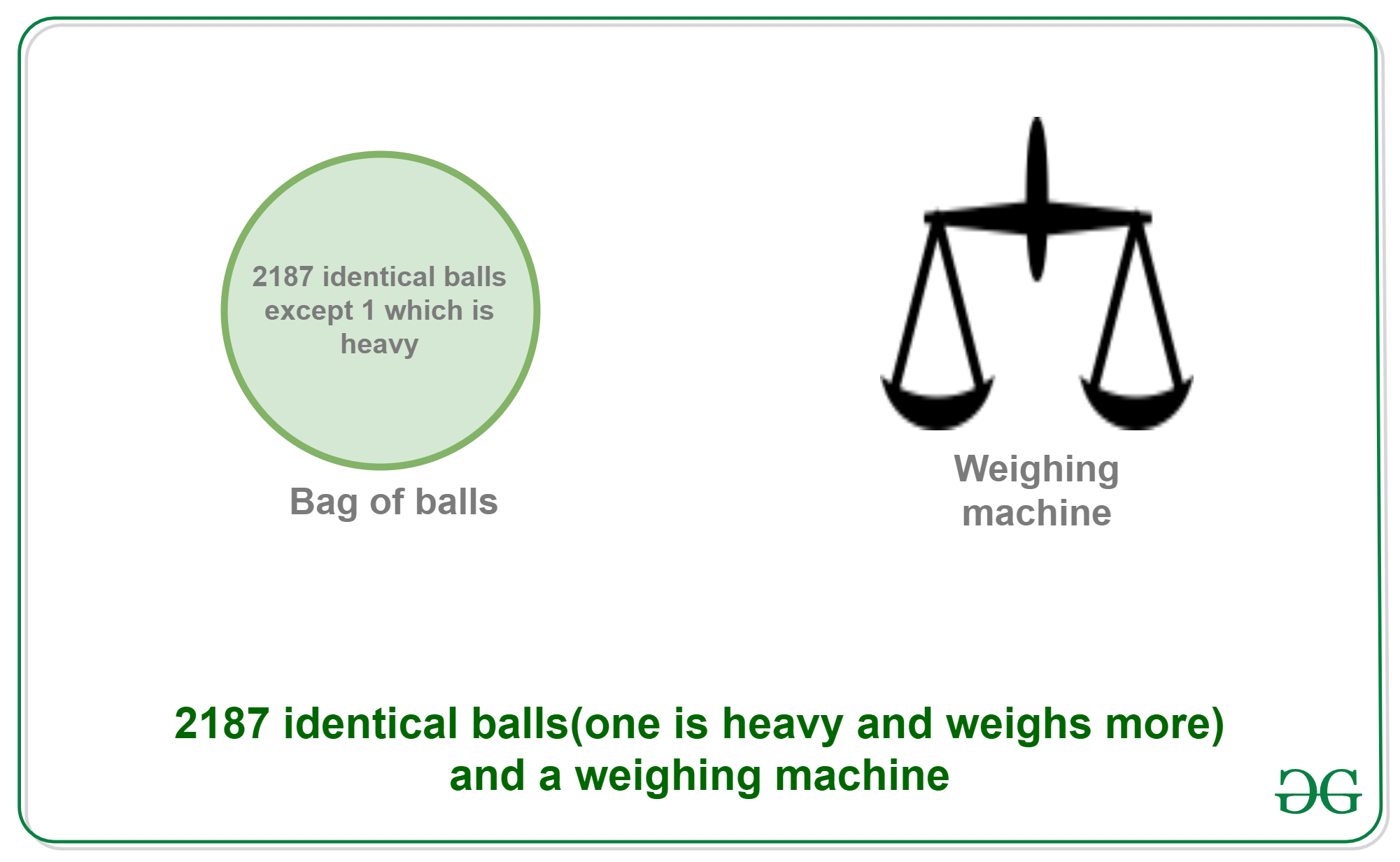Solution: The minimum amount of measurements needed to be done will be equal to 7 times.
2187 = 3^7

1. Step 1: Divide the 2187 balls into 3 equal groups of 729 each, let’s say C1, C2 and C3. Put C1 on one side of the weighing machine and C2 on the other. This can give rise to 3 conditions:
• Condition 1: C1 equals C2, which means C3 has the heaviest ball
• Condition 2: C1 < C2, which means C2 has the heaviest ball.
• Condition 3: C1 > C2, which means C1 has the heaviest ball.

Let’s suppose Condition 1 follows up, and C3 has the heaviest ball.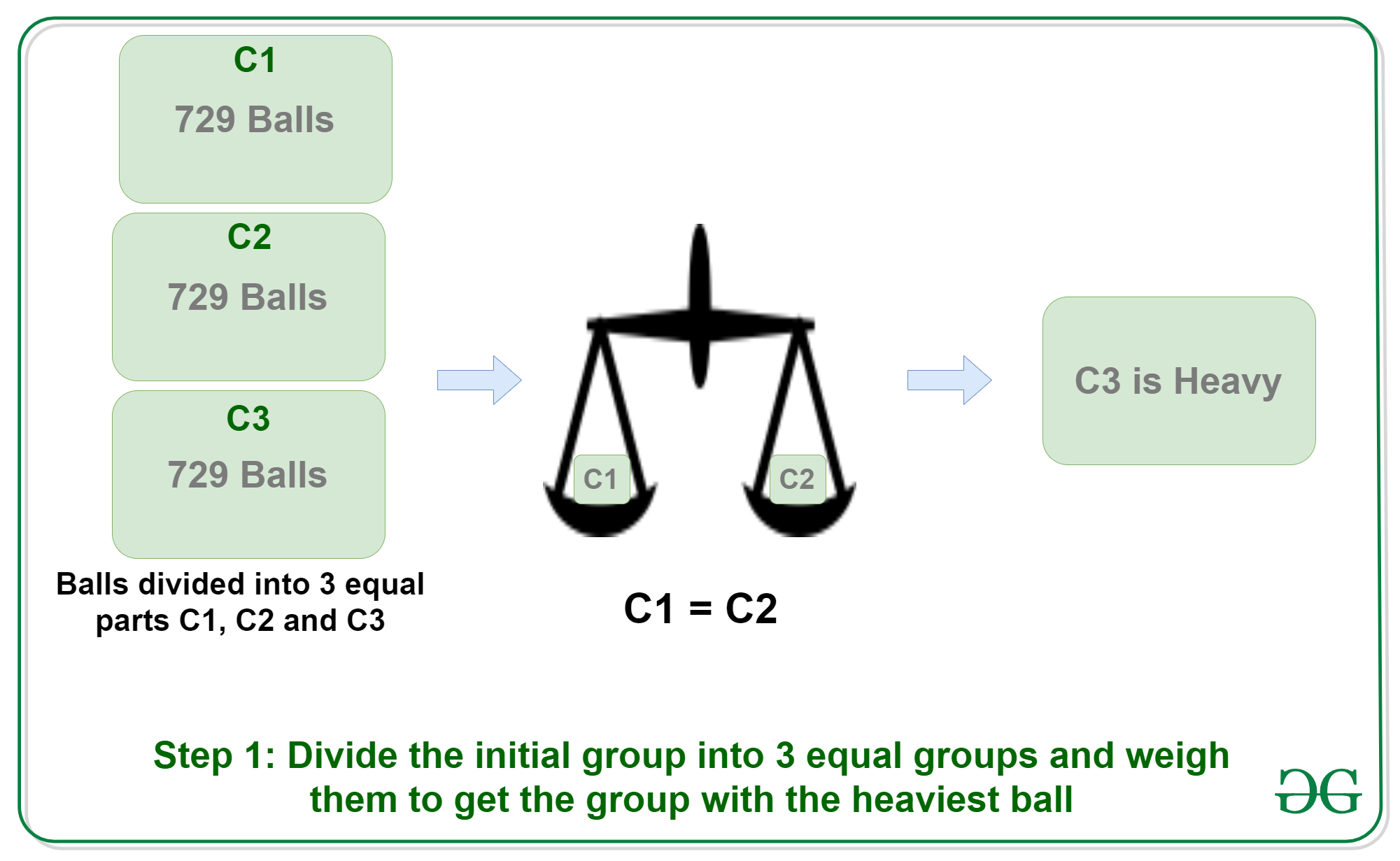2. Step 2: Now divide C3 again into 3 equal groups of 243 each, naming them again C1, C2 and C3. Put C1 on one side of the weighing machine and C2 on the other. This can give rise to 3 conditions:
• Condition 1: C1 equals C2, which means C3 has the heaviest ball
• Condition 2: C1 < C2, which means C2 has the heaviest ball.
• Condition 3: C1 > C2, which means C1 has the heaviest ball.

Let’s suppose Condition 2 follows up, and C2 has the heaviest ball.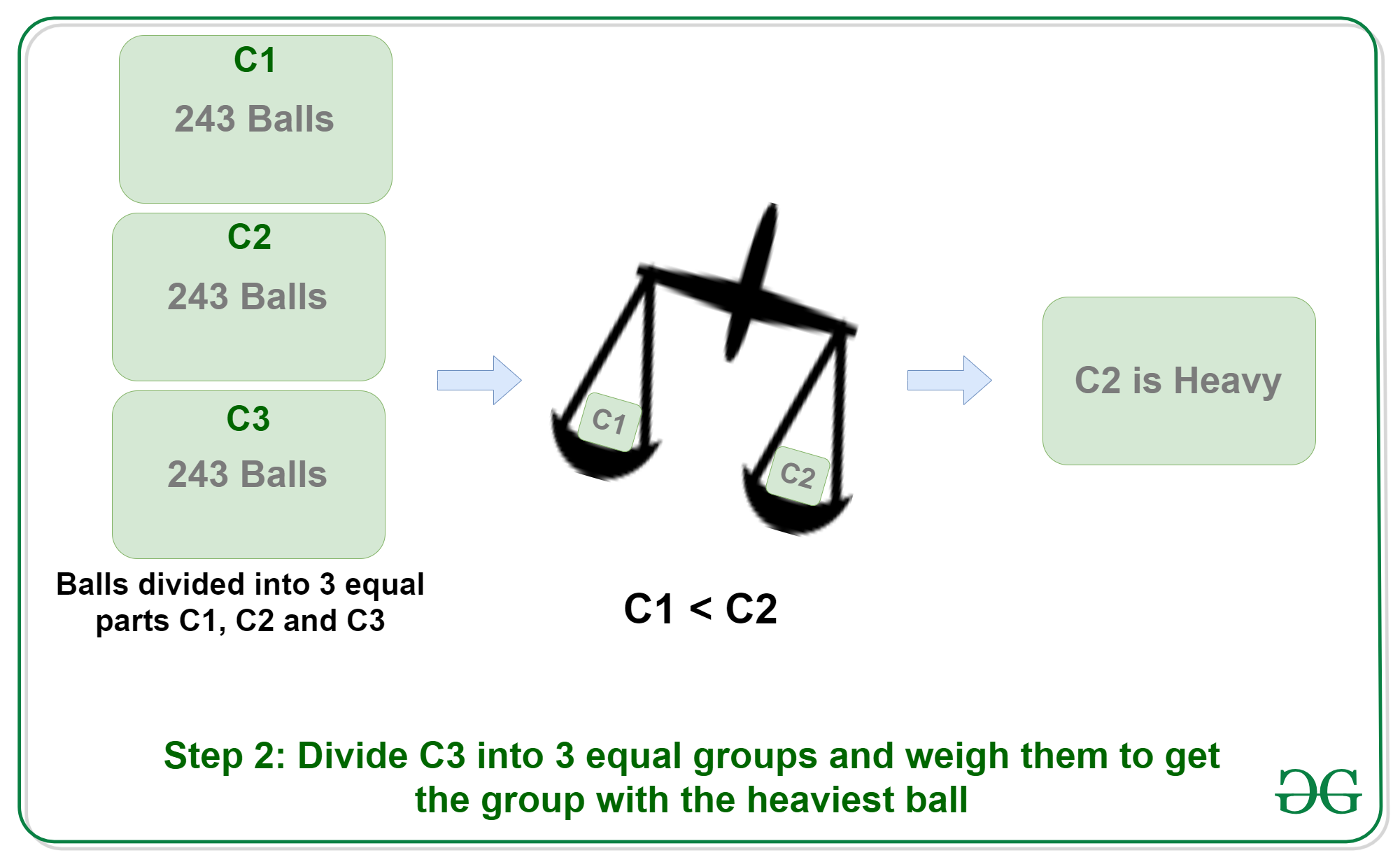3. Step 3: Now divide C2 again into 3 equal groups of 81 each, naming them again C1, C2 and C3. Put C1 on one side of the weighing machine and C2 on the other. This can give rise to 3 conditions:
• Condition 1: C1 equals C2, which means C3 has the heaviest ball
• Condition 2: C1 < C2, which means C2 has the heaviest ball.
• Condition 3: C1 > C2, which means C1 has the heaviest ball.

Let’s suppose Condition 3 follows up, and C1 has the heaviest ball.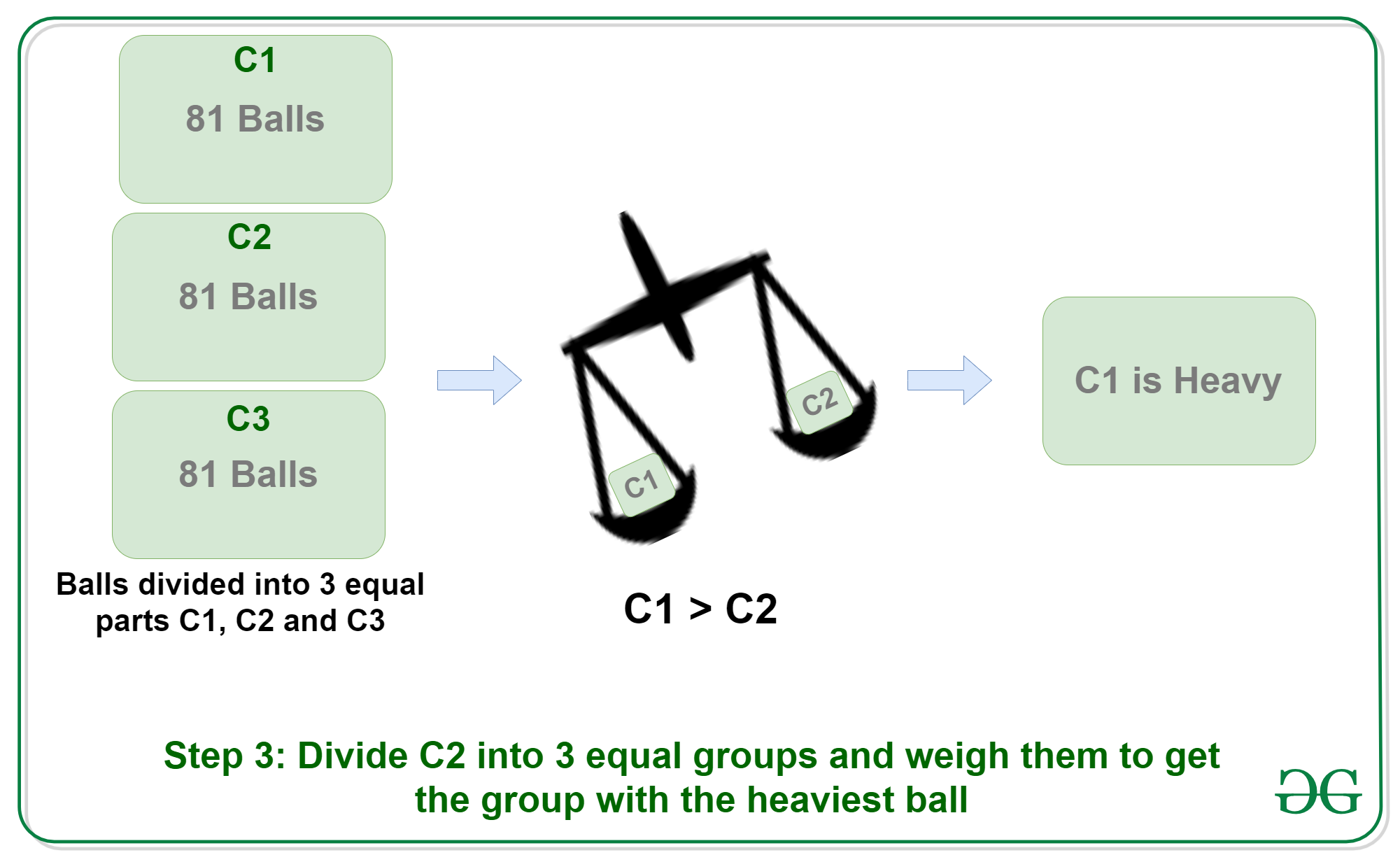4. Step 4: Now divide C1 again into 3 equal groups of 27 each, naming them again C1, C2 and C3. Put C1 on one side of the weighing machine and C2 on the other. This can give rise to 3 conditions:
• Condition 1: C1 equals C2, which means C3 has the heaviest ball
• Condition 2: C1 < C2, which means C2 has the heaviest ball.
• Condition 3: C1 > C2, which means C1 has the heaviest ball.

Let’s suppose Condition 3 follows up, and C1 has the heaviest ball.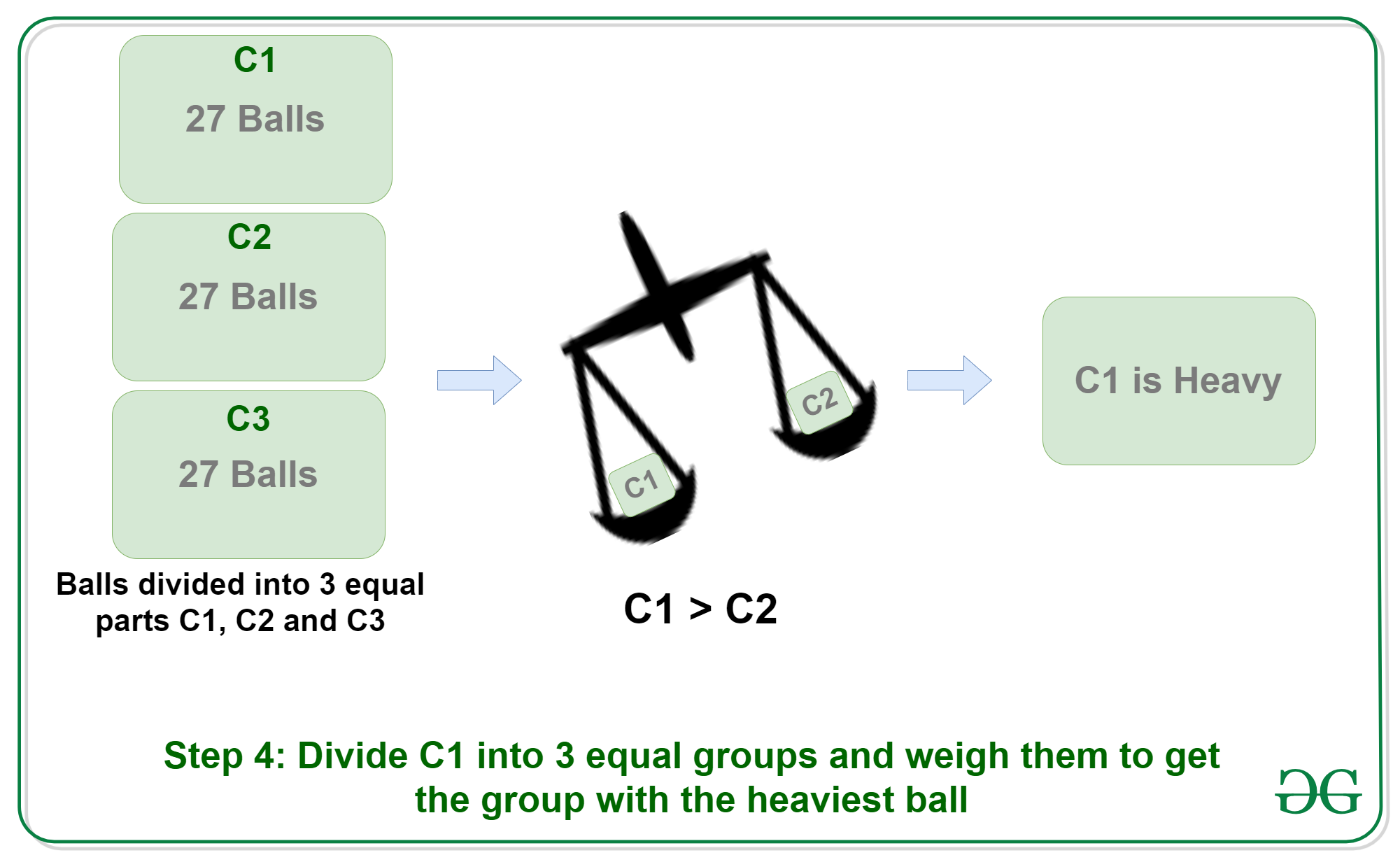5. Step 5: Now divide C1 again into 3 equal groups of 9 each, naming them again C1, C2 and C3. Put C1 on one side of the weighing machine and C2 on the other. This can give rise to 3 conditions:
• Condition 1: C1 equals C2, which means C3 has the heaviest ball
• Condition 2: C1 < C2, which means C2 has the heaviest ball.
• Condition 3: C1 > C2, which means C1 has the heaviest ball.

Let’s suppose Condition 1 follows up, and C3 has the heaviest ball.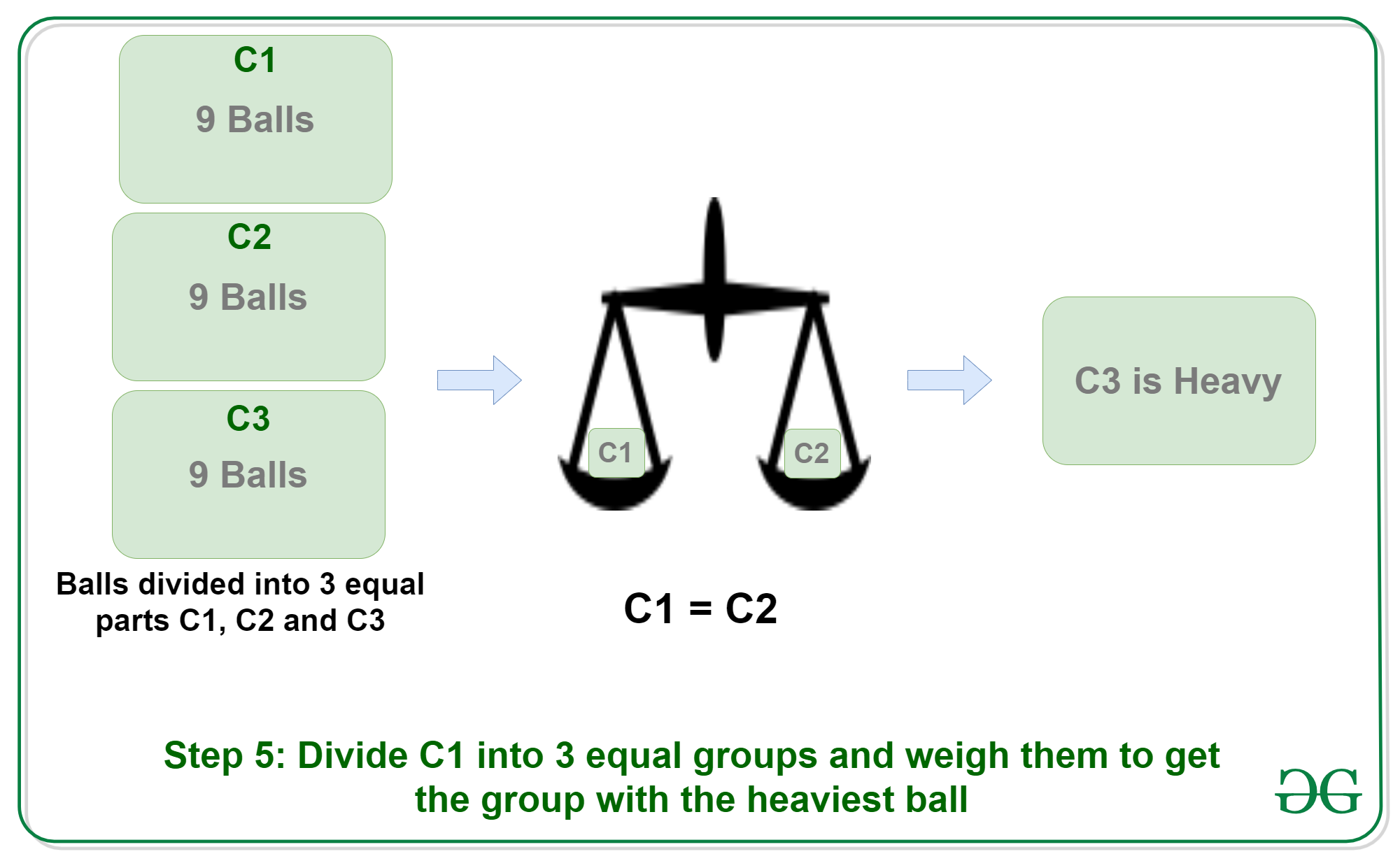6. Step 6: Now divide C3 again into 3 equal groups of 3 each, naming them again C1, C2 and C3. Put C1 on one side of the weighing machine and C2 on the other. This can give rise to 3 conditions:
• Condition 1: C1 equals C2, which means C3 has the heaviest ball
• Condition 2: C1 < C2, which means C2 has the heaviest ball.
• Condition 3: C1 > C2, which means C1 has the heaviest ball.

Let’s suppose Condition 1 follows up, and C3 has the heaviest ball.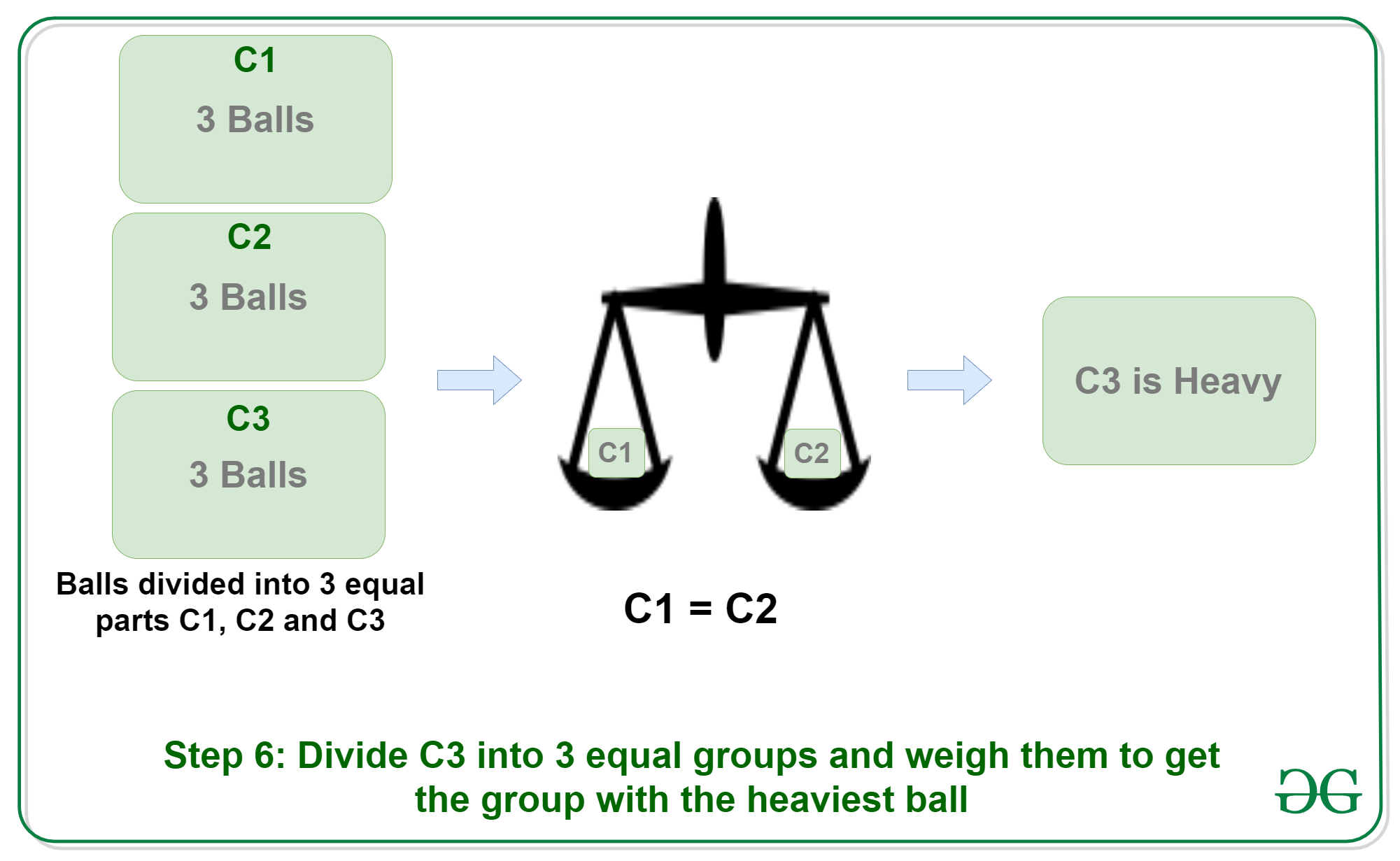7. Step 7: Now finally divide C3 which has 3 balls into group of 1, naming them again B1, B2 and B3. Put B1 on one side of the weighing machine and B2 on the other. This can give rise to 3 conditions:
• Condition 1: B1 equals B2, which means B3 has the heaviest ball
• Condition 2: B1 < B2, which means B2 has the heaviest ball.
• Condition 3: B1 > B2, which means B1 has the heaviest ball.

Let’s suppose Condition 1 follows up, then B3 is the heaviest ball we were looking for.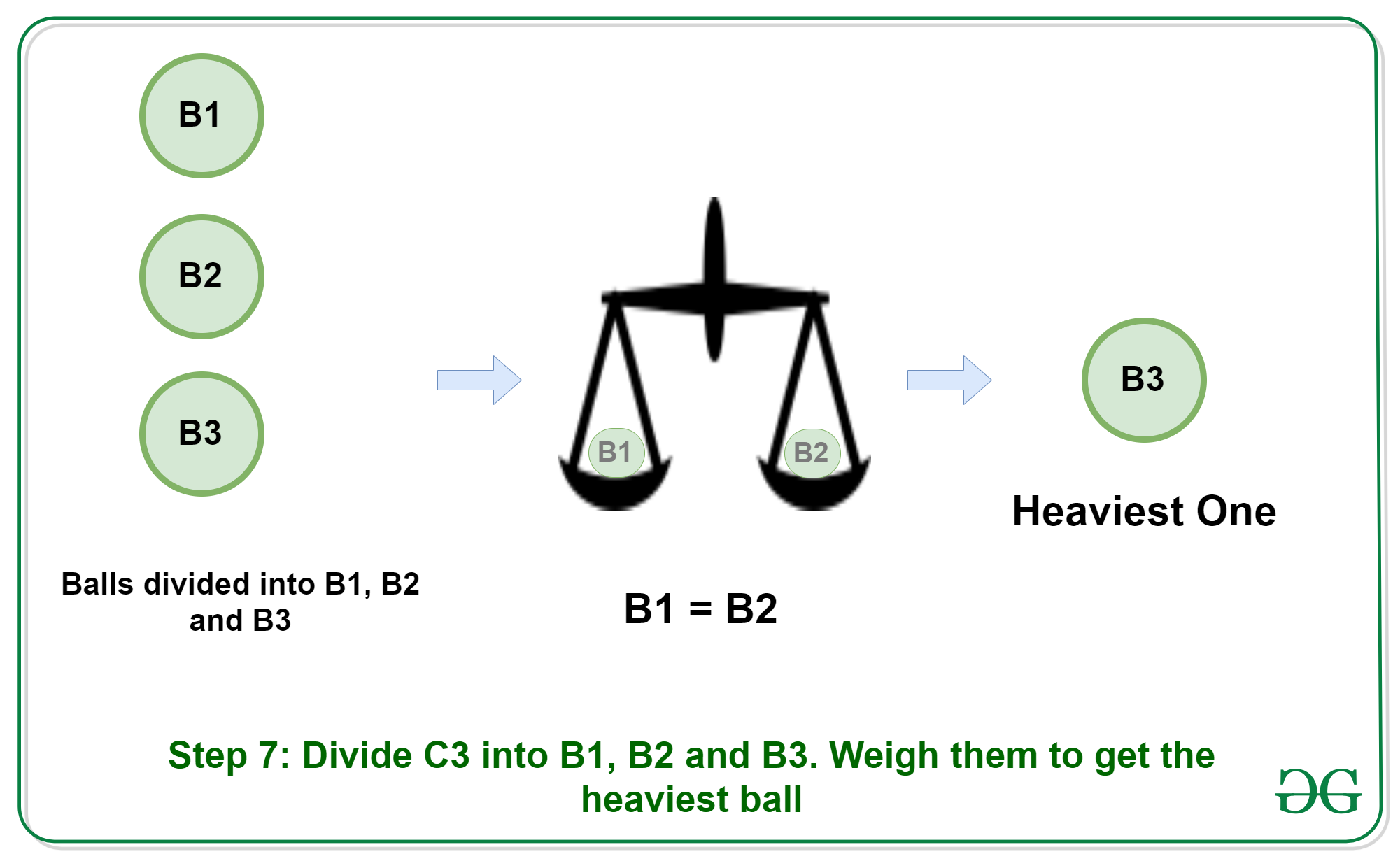So, in all, it takes 7 steps to reach to the heaviest ball.

This article is contributed by Shubham Singh. If you like GeeksforGeeks and would like to contribute, you can also write an article using contribute.geeksforgeeks.org or mail your article to contribute@geeksforgeeks.org. See your article appearing on the GeeksforGeeks main page and help other Geeks.